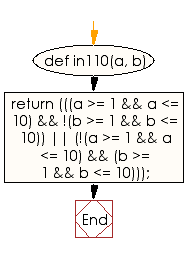﻿ Ruby Basic exercises: Check three numbers into the range - w3resource# Ruby Basic Exercises: Check three numbers into the range

## Ruby Basic: Exercise-10 with Solution

10. Write a Ruby program to check three numbers and return true if one or the other is small, but not both. A number is called "small" if it is in the range 1..10 inclusive.

Ruby Code:

``````def in110(a, b)
return (((a >= 1 && a <= 10) && !(b >= 1 && b <= 10)) ||
(!(a >= 1 && a <= 10) && (b >= 1 && b <= 10)));
end
print in110(1, 12),"\n"
print in110(10, 15),"\n"
print in110(11, 25),"\n"
``````

Output:

```true
true
false
```

Flowchart:Ruby Code Editor: